# Remainder of an integer

(diff) ← Older revision | Latest revision (diff) | Newer revision → (diff)modulo, residue ofmoduloAny integerwhich is congruent tomodulo(cf. Congruence). Letbe the remainder of division ofby some integer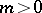,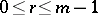; then the residueof the numbermodulowill have the form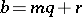, whereis some integer. The residue corresponding to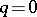is equal toand is called the least non-negative residue of. The smallest (in absolute value) residueis called the absolutely smallest residue of. If, then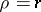; if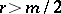, then; finally, ifis even and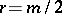, eitherormay be taken as.

A system consisting ofintegers each one of which is the residue of one and only one of the numbersis called a complete system of residues modulo. The smallest non-negative residues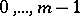or the absolutely smallest residues are the complete systems of residues which are most frequently used.

A power residue of degreemodulo,, is any integer, coprime with, for which the congruenceis solvable. If this congruence is not solvable,is called a power non-residue of degreemodulo. In particular, if, the residues or non-residues are called quadratic; if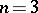, they are called cubic; if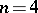, they are called biquadratic (see also Power residue).

How to Cite This Entry:
Remainder of an integer. Encyclopedia of Mathematics. URL: http://encyclopediaofmath.org/index.php?title=Remainder_of_an_integer&oldid=13893
This article was adapted from an original article by S.A. Stepanov (originator), which appeared in Encyclopedia of Mathematics - ISBN 1402006098. See original article